# Level 4

In R the basic trigonometry functions use Radians instead of Degrees. The relationship between radians and degrees is the following:

Angle in radians = Angle in degrees * (pi/180)

Write the code to compute the value of 45 degrees in radians.

Now… To build our first function! If you want to a) subtract two numbers and b) obtain the absolute value of the operation done in a); you could do so with a few lines of code like:

Number_1 = -168
Number_2 = -126
Result = Number_1 - Number_2
Final_result = abs(Result)

Every time you would want to repeat this operation but with different numbers you would have to use the code above but changing the values of Number_1 and Number_2.

Number_1 = 1191
Number_2 = 1233
Result = Number_1 - Number_2
Final_result = abs(Result)
print(x=Final_result)
##  42

If you write a function to do it however, if you want to repeat the operation you can do so with a single line of code later on. To do the same operation as the one described above using a function, you could do:

My_function = function(Number_1, Number_2){
Result = Number_1 - Number_2
Final_result = abs(Result)
return(Final_result)
}

return(abs(Number_1 - Number_2))
}

Both functions do the same, but the cool thing is that after doing this, you can obtain the same results as above with a single line of code for each of the examples as:

My_final_result_1 = My_function(Number_1=-168, Number_2=-126)
My_final_result_2 = My_function(Number_1=1191, Number_2=1233)
print(x=My_final_result_1)
##  42
print(x=My_final_result_2)
##  42

Writing our first function in high-school math notation gives us: $$f(x, y) = |g(x, y)|$$ $$g(x, y) = x - y$$

Or if we simplify: $$f(x,y) = |x-y|$$

There are a couple of things that you should know about functions. Let us use kitchen robots again as an example. In R, these kitchen robots clean after themselves, that is, if you give it the ingredients, they’ll give you a meal and afterwars, inside there won’t be anything, just the instructions to execute the recipe. Also if you don’t provide the correct ingredients, the robot will not know what to do. If your robot makes pasta and if you provide as ingredients rice and a shoe it will probably stop working.

## TO-DO

Write a function that takes in degrees and gives back/returns radians.

Use it to compute the radian values of a 90, 45 and 0 degree angles.

## TO-DO

Use these basic trigonometry functions (they only accept Radians):

• cos(x) - cosine;
• sin(x) - sine;
• tan(x) - tangent

and write the code to compute the sine, cosine and tangent of a 90 degree angle and check the result.

# Level 5

At this point you should be able to start working on real problems, so let’s do just that!

gene_data = read.csv("gene_expression_data.csv")

### Help

To check how the read.csv function works check the read.csv page by typing ?read.csv in the console.

?read.csv

This is just a simple description. The help page has much more useful information!

read.table(file, header = FALSE, sep = "", quote = "\"'",
dec = ".", numerals = c("allow.loss", "warn.loss", "no.loss"),
row.names, col.names, as.is = !stringsAsFactors,
na.strings = "NA", colClasses = NA, nrows = -1,
skip = 0, check.names = TRUE, fill = !blank.lines.skip,
strip.white = FALSE, blank.lines.skip = TRUE,
comment.char = "#",
allowEscapes = FALSE, flush = FALSE,
stringsAsFactors = default.stringsAsFactors(),
fileEncoding = "", encoding = "unknown", text, skipNul = FALSE)

dec = ".", fill = TRUE, comment.char = "", ...)

dec = ",", fill = TRUE, comment.char = "", ...)

dec = ".", fill = TRUE, comment.char = "", ...)

dec = ",", fill = TRUE, comment.char = "", ...)

### Check what is inside

We can quickly check what is inside this object with a few commands.

dim(gene_data)     # gives us the dimensions of the table
##  305   4
head(gene_data)    # shows us the first 6 rows of the table
##           X     geneA     geneB   cell_type
## 1 FIBRO-9DW 0.7542634 0.9423952 FIBROBLASTS
## 2 FIBRO-TPA 0.7476058 0.9396038 FIBROBLASTS
## 3 FIBRO-TYL 0.7476938 0.9457088 FIBROBLASTS
## 4 FIBRO-4LI 0.7667875 0.9727001 FIBROBLASTS
## 5 FIBRO-7CJ 0.7712265 0.9645454 FIBROBLASTS
## 6 FIBRO-50S 0.7625049 0.9724643 FIBROBLASTS
summary(gene_data) # shows us information about the different columns of the table
##       X                 geneA            geneB         cell_type
##  Length:305         Min.   :0.7318   Min.   :0.9116   Length:305
##  Class :character   1st Qu.:0.7630   1st Qu.:0.9560   Class :character
##  Mode  :character   Median :1.3015   Median :0.9798   Mode  :character
##                     Mean   :1.0982   Mean   :0.9822
##                     3rd Qu.:1.3158   3rd Qu.:0.9926
##                     Max.   :1.3348   Max.   :1.2552

With the previous commands we can already say quite a few things about the data we have loaded. It has 305 rows and 4 columns. The 1st column is an experiment identifier, the 2nd and 3rd columns is the gene expression information about two genes (geneA and geneB). Finally, the 4th column is named “cell_type”, which contains the cell type associated with each different sample.

We can use the command “table” on the column “cell_type” of the data we loaded to obtain a count of each of the different cell types present in this data in an easy to read form.

If you remember, from the previous exercises and the lecture, this is done with “”. Further since we are looking at some sort of matrix and we want a column, we know that we should place the name of the column after a comma (data[,“name_of_column”]).

count_cell_types = table(gene_data[,"cell_type"])
count_cell_types
##
## BLOOD.CELLS FIBROBLASTS        IPSC
##         184         108          13

### Help

For detailed help use ?NAME_OF_FUNCTION.

?dim
?table
?summary

dim

dim(x)
dim(x) <- value

head(x, ...)
## Default S3 method:

## S3 method for class 'matrix'
## NB: The methods for 'data.frame' and 'array'  are identical to the 'matrix' one

## S3 method for class 'ftable'
## S3 method for class 'function'

tail(x, ...)
## Default S3 method:
tail(x, n = 6L, keepnums = FALSE, addrownums, ...)
## S3 method for class 'matrix'
tail(x, n = 6L, keepnums = TRUE, addrownums, ...) # exported as tail.matrix()
## NB: The methods for 'data.frame', 'array', and 'table'
##     are identical to the  'matrix'  one

## S3 method for class 'ftable'
tail(x, n = 6L, keepnums = FALSE, addrownums, ...)
## S3 method for class 'function'
tail(x, n = 6L, ...)

table

table(...,
exclude = if (useNA == "no") c(NA, NaN),
useNA = c("no", "ifany", "always"),
dnn = list.names(...), deparse.level = 1)

as.table(x, ...)
is.table(x)

## S3 method for class 'table'
as.data.frame(x, row.names = NULL, ...,
responseName = "Freq", stringsAsFactors = TRUE,
sep = "", base = list(LETTERS))

summary

summary(object, ...)

## Default S3 method:
summary(object, ..., digits, quantile.type = 7)
## S3 method for class 'data.frame'
summary(object, maxsum = 7,
digits = max(3, getOption("digits")-3), ...)

## S3 method for class 'factor'
summary(object, maxsum = 100, ...)

## S3 method for class 'matrix'
summary(object, ...)

## S3 method for class 'summaryDefault'
format(x, digits = max(3L, getOption("digits") - 3L), ...)
## S3 method for class 'summaryDefault'
print(x, digits = max(3L, getOption("digits") - 3L), ...)

With the information gathered through the few commands we ran, we can say that:

• the table has the gene expression values for genes A and B for 305 different samples from 3 different cell types.
• there are 184 blood cells samples, 108 fibroblasts and 13 IPSCs in this dataset.

### Plotting

There are several different functions that you can use to analyse this data. For example we can use the count data that we generated above with the table function to quickly generate a pie chart or bar plot with the number of samples per cell type in our data.

pie(count_cell_types)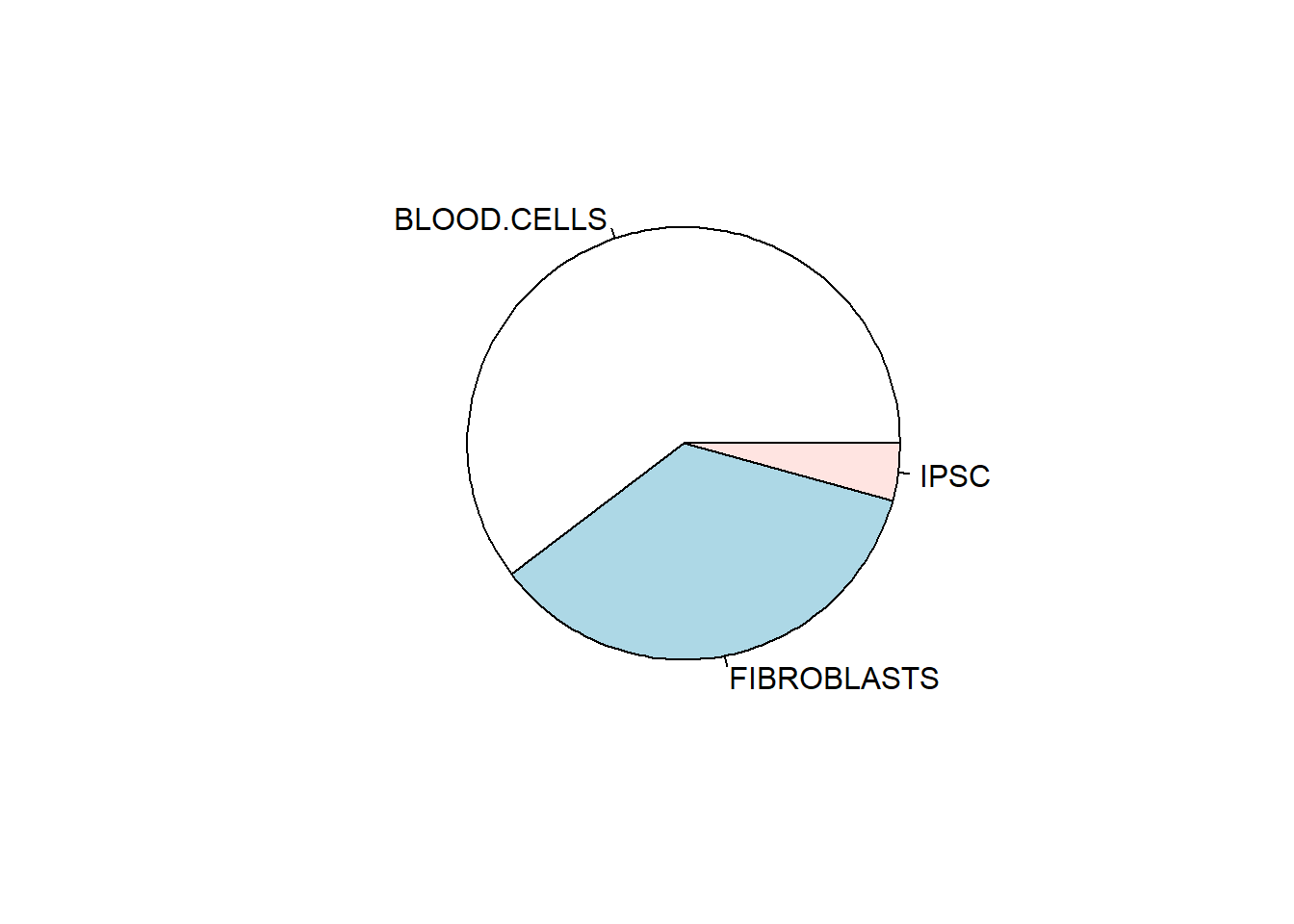barplot(count_cell_types)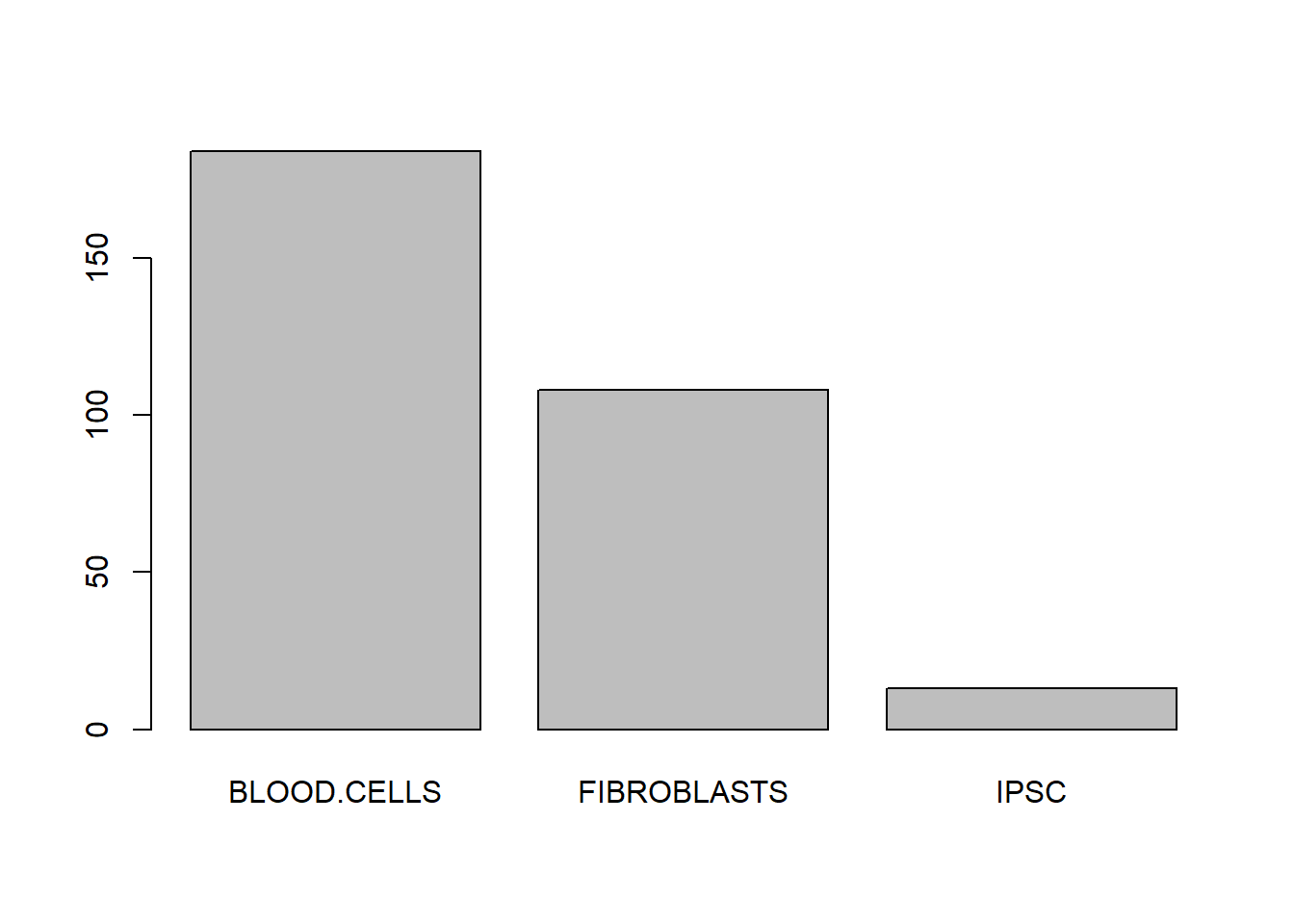These are fine but we can define our own colours that we can use here and throughout the whole exercise to keep the plots consistent with each other. R has a number of predefined colours that you can call by name (e.g. “red”,“black”,“green”) and which you can check in this link. You can also use a hexadecimal colour code, commonly called hexcolor, to define over 16 million colours. In “hexcolor”, red can be “#ff0000”, black is “#000000” and green can be “#42853c”. Hexcolour codes always start with a “#” symbol followed by 6 alfanumerical characters.

In this exercise we will use the colors pre-defined in R but feel free to change these to colours you like. Lets define cell_colours and use it throughout the exercise.

cell_colours = c("red", "blue", "green")

Lets repeat the previous plots but with colours.

pie(count_cell_types, col=cell_colours)     # the col argument defines the color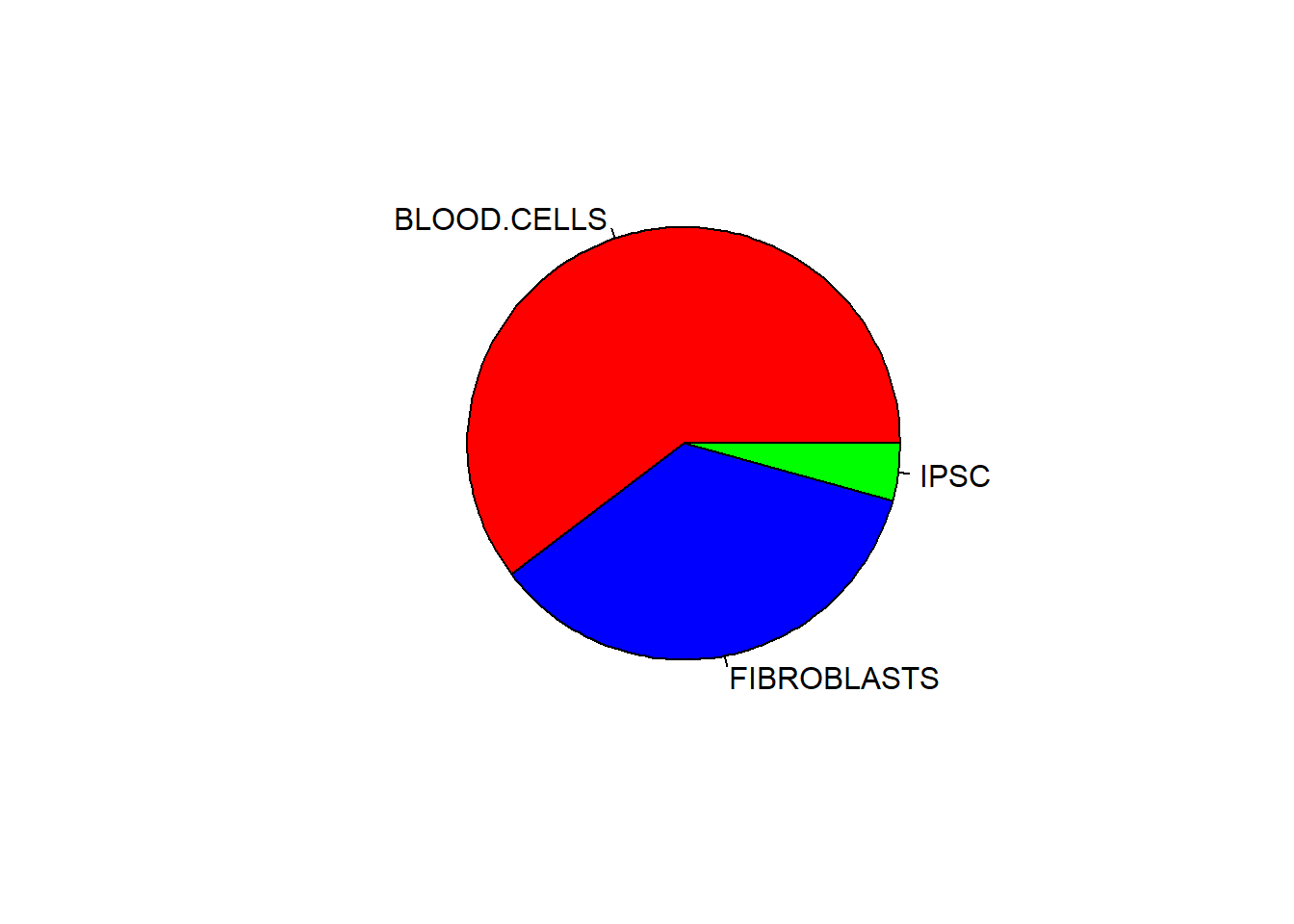barplot(count_cell_types, col=cell_colours) # the col argument defines the color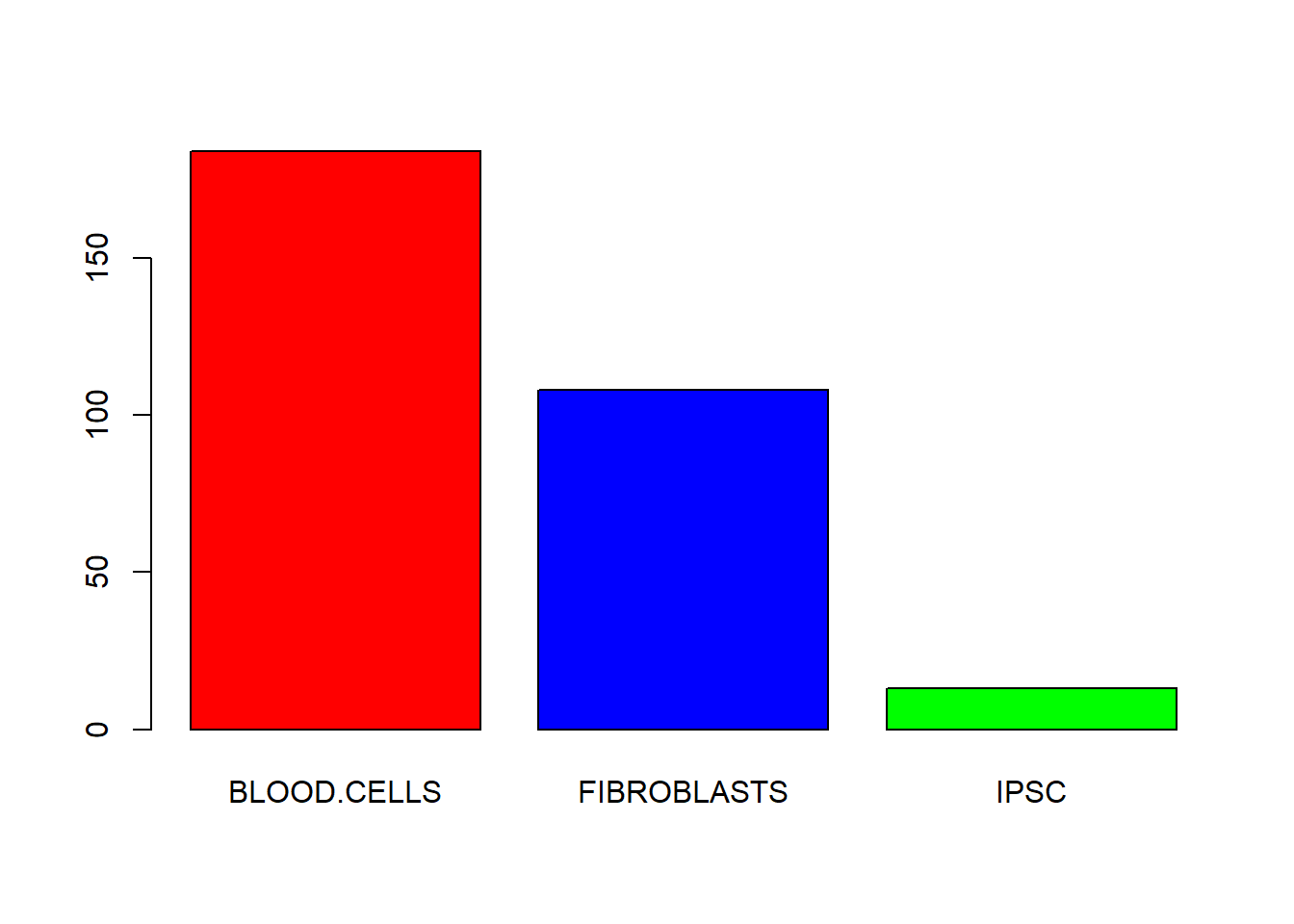A scatter plot, where we show the expression of the two genes for all samples, provides the best vizualisation for this dataset. To do this, we can use the function plot. It has many arguments and you should check its help page. To start with we will just use the x and y arguments.

plot(
x=gene_data[,"geneA"], # data in the x axis
y=gene_data[,"geneB"]  # data in the y axis
)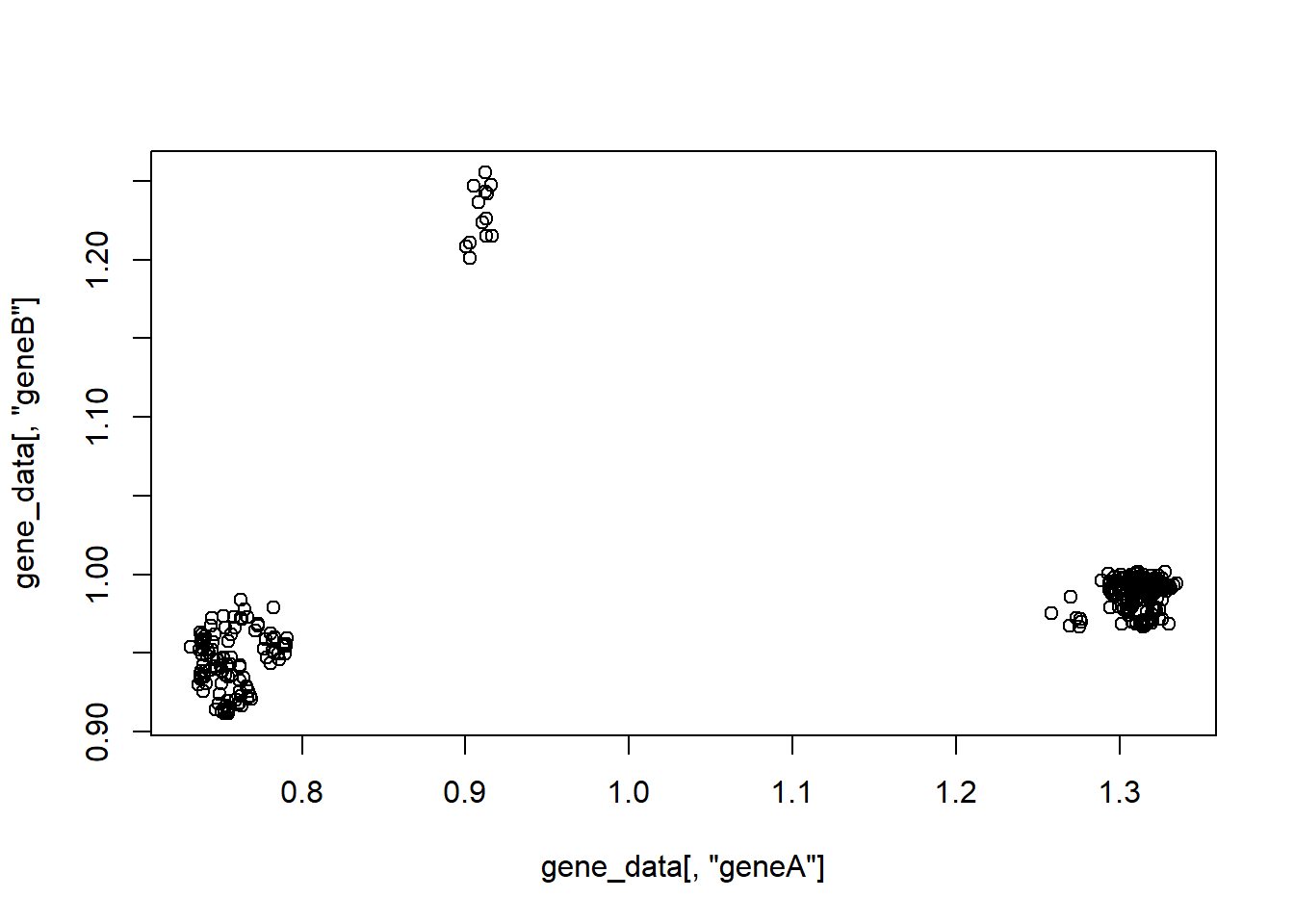### Help

For more details do ?plot, ?pie, ?barplot.

## Default S3 method:
plot(x, y = NULL, type = "p",  xlim = NULL, ylim = NULL,
log = "", main = NULL, sub = NULL, xlab = NULL, ylab = NULL,
ann = par("ann"), axes = TRUE, frame.plot = axes,
panel.first = NULL, panel.last = NULL, asp = NA,
xgap.axis = NA, ygap.axis = NA,
...)
pie(x, labels = names(x), edges = 200, radius = 0.8,
clockwise = FALSE, init.angle = if(clockwise) 90 else 0,
density = NULL, angle = 45, col = NULL, border = NULL,
lty = NULL, main = NULL, ...)
barplot(height, ...)

## Default S3 method:
barplot(height, width = 1, space = NULL,
names.arg = NULL, legend.text = NULL, beside = FALSE,
horiz = FALSE, density = NULL, angle = 45,
col = NULL, border = par("fg"),
main = NULL, sub = NULL, xlab = NULL, ylab = NULL,
xlim = NULL, ylim = NULL, xpd = TRUE, log = "",
axes = TRUE, axisnames = TRUE,
cex.axis = par("cex.axis"), cex.names = par("cex.axis"),
inside = TRUE, plot = TRUE, axis.lty = 0, offset = 0,
add = FALSE, ann = !add && par("ann"), args.legend = NULL, ...)

## S3 method for class 'formula'
barplot(formula, data, subset, na.action,
horiz = FALSE, xlab = NULL, ylab = NULL, ...)

This shows that samples group within the expression space, but we do not have information about the cell types. We can add visual queues to vizualise the distribution of distinct cell types by using colour.

We want to colour each sample by its cell type. To do this we will use the “cell_type” column in our table. We will save this column to a variable and subsequently transform it to a factor. Factors are just easier to work with in this context.

cell_type_data = as.factor(gene_data[,"cell_type"])

plot(
x=gene_data[,"geneA"], # data in the x axis
y=gene_data[,"geneB"], # data in the y axis
col=cell_type_data     # colour
)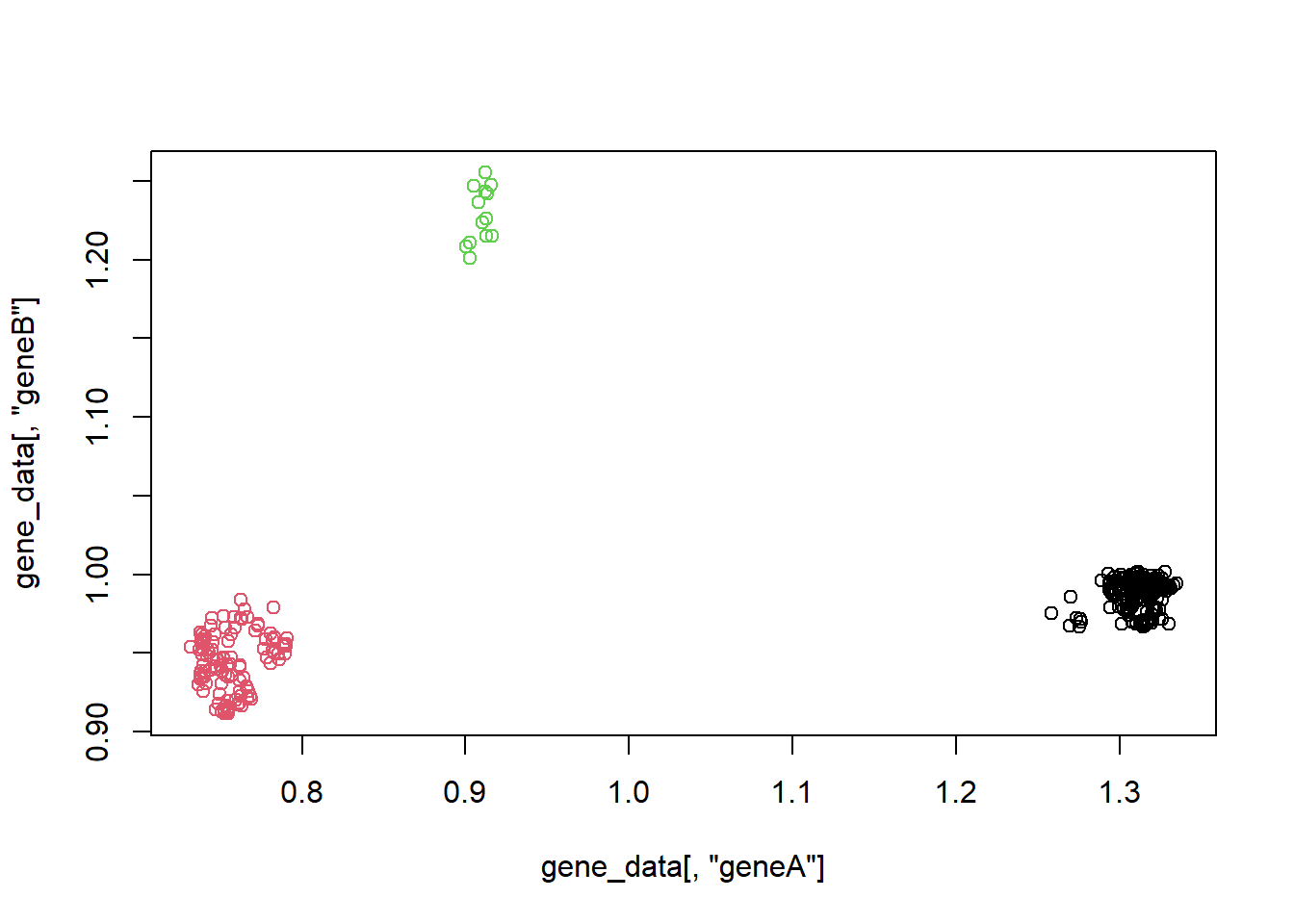Because of how factors work we can use them to subset the colour vector we created (cell_colours) with the categories of the factor itself (the different cell types). This makes it so that each of the cell types has the colour we want for it.

plot(
x=gene_data[,"geneA"], # data in the x axis
y=gene_data[,"geneB"], # data in the y axis
col=cell_colours[cell_type_data]     # colour
)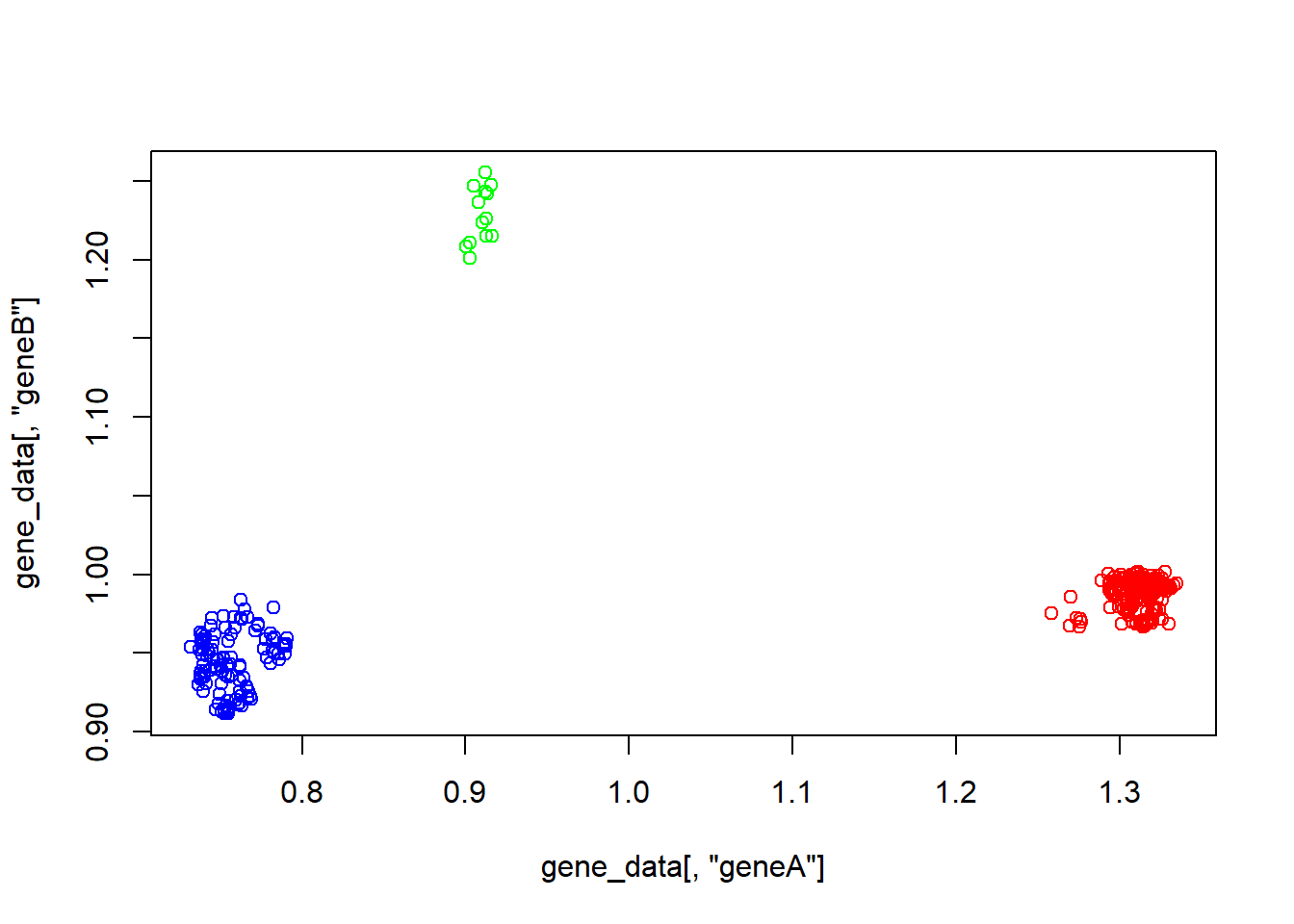We can clearly see now that distinct cell types have distinct expression values. We can improve this by using dots instead of circles in the plot. There is one argument in the plot function that does this. The argument is called pch and depending on the number that we provide it with, we will have a different symbol in the plot. You can see the available symbols below.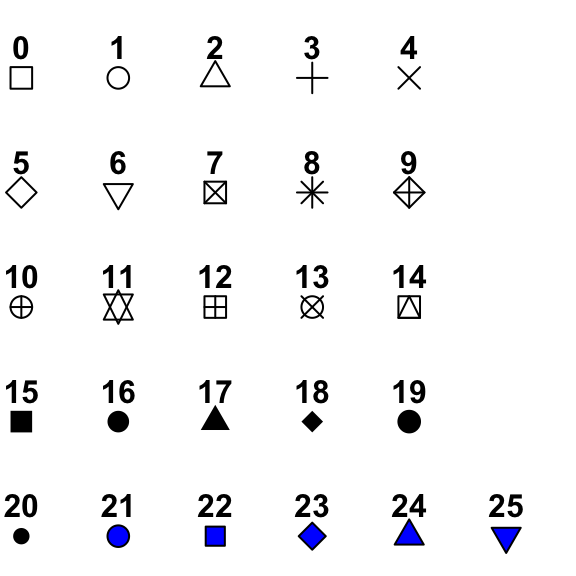plot(
x=gene_data[,"geneA"], # data in the x axis
y=gene_data[,"geneB"], # data in the y axis
col=cell_colours[cell_type_data],    # sample colour
pch=19                 # symbol shape
)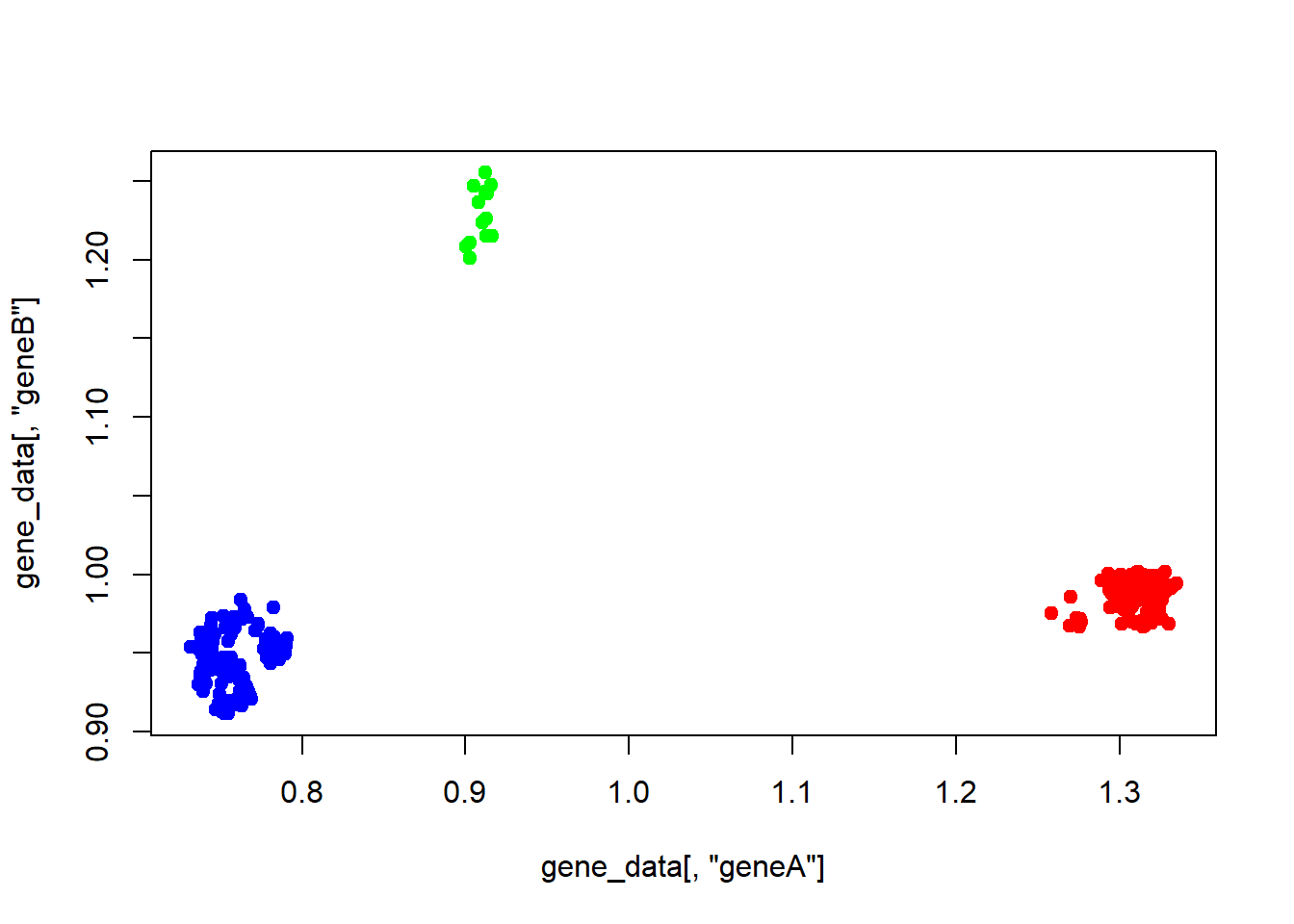Now it is starting to look good! We should add a title and perhaps fix the name of the labels in the x and y axis. We can do this with the arguments main, xlab and ylab.

plot(
x=gene_data[,"geneA"],             # data in the x axis
y=gene_data[,"geneB"],             # data in the y axis
col=cell_colours[cell_type_data],  # sample colour
pch=19,                            # symbol shape
main="Gene A vs B scatterplot",    # title of the plot
ylab="Gene B expression",          # y axis label
xlab="Gene A expression"           # x axis label
)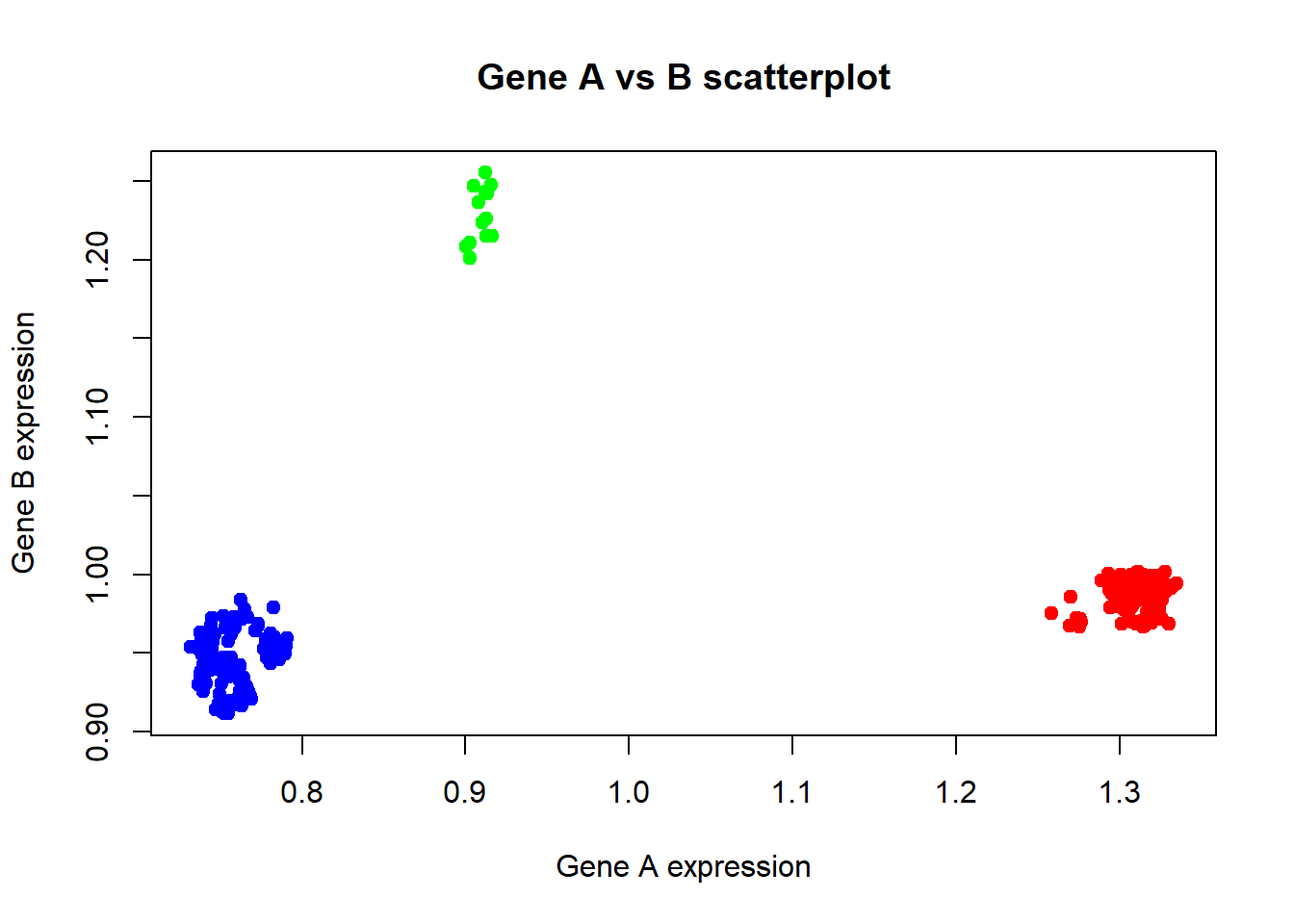Now this is already a pretty good looking plot. We are only missing a legend now. To produce a legend we need to call the legend function right after we called the plot function. Check the help page of the legend function to understand its arguments.

plot(
x=gene_data[,"geneA"],             # data in the x axis
y=gene_data[,"geneB"],             # data in the y axis
col=cell_colours[cell_type_data],  # sample colour
pch=19,                            # symbol shape
main="Gene A vs B scatterplot",    # title of the plot
ylab="Gene B expression",          # y axis label
xlab="Gene A expression"           # x axis label
)
legend(
x="topright",
legend=levels(cell_type_data),
col=cell_colours,
pch=19
)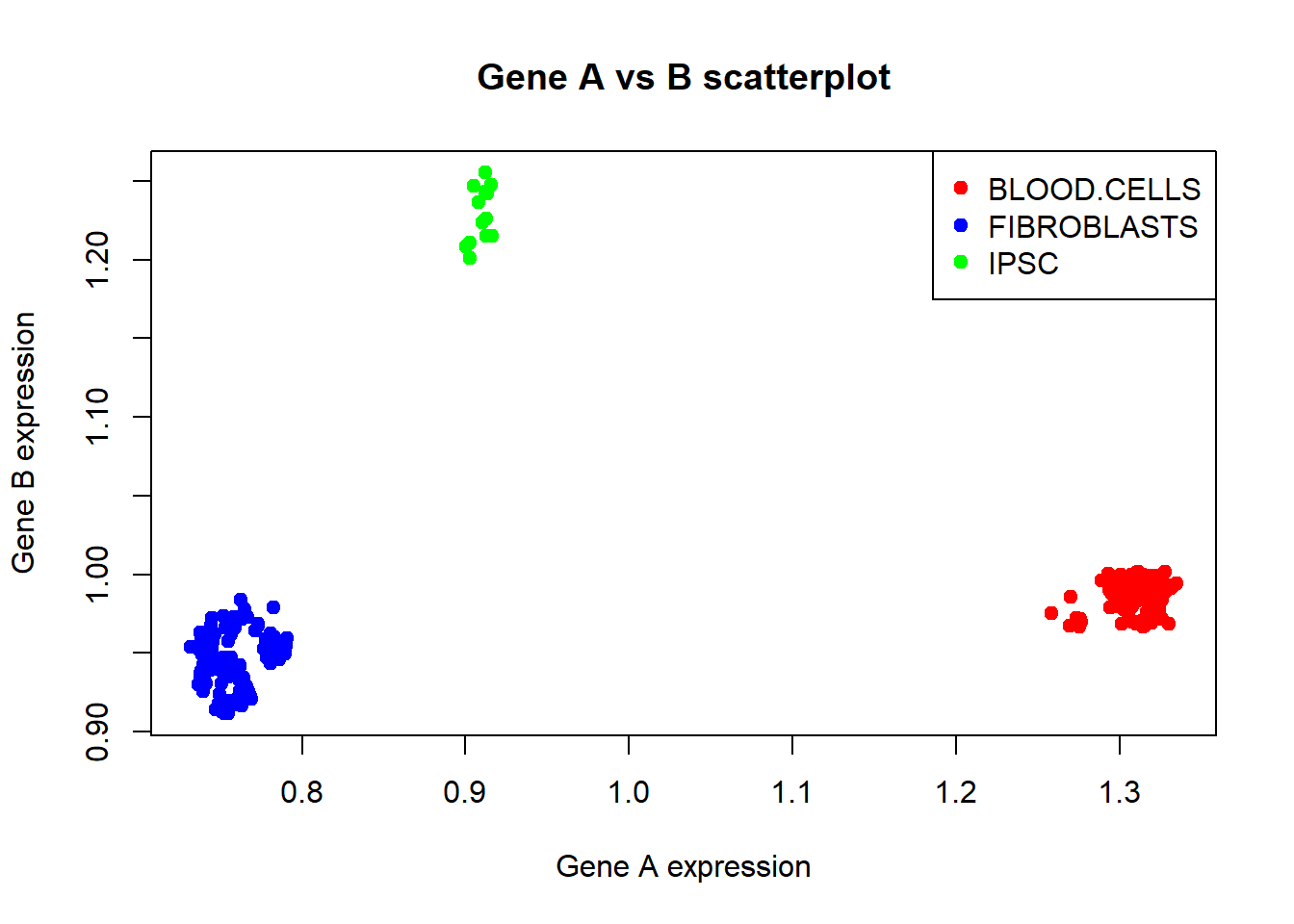### Help

For more details do ?legend

legend(x, y = NULL, legend, fill = NULL, col = par("col"),
border = "black", lty, lwd, pch,
angle = 45, density = NULL, bty = "o", bg = par("bg"),
box.lwd = par("lwd"), box.lty = par("lty"), box.col = par("fg"),
pt.bg = NA, cex = 1, pt.cex = cex, pt.lwd = lwd,
xjust = 0, yjust = 1, x.intersp = 1, y.intersp = 1,
adj = c(0, 0.5), text.width = NULL, text.col = par("col"),
text.font = NULL, merge = do.lines && has.pch, trace = FALSE,
plot = TRUE, ncol = 1, horiz = FALSE, title = NULL,
inset = 0, xpd, title.col = text.col, title.adj = 0.5,
seg.len = 2)

## More plots

Depending how your data looks like you might have to transform it in order to plot it.

Further, as you have probably noticed by now if you have been checking the help pages, the same (plotting) functions can take completely different arguments.

One of such functions is the boxplot function which, you guessed it, produces boxplots.

A boxplot is a plot that displays in a concise and standardized way, information about the distribution of our data in a quick, easy and understandable way.

It focus on 5 key values, “minimum”, first quartile (Q1), median, third quartile (Q3), and “maximum”.

It is of great use when you are trying to check how values (gene expression) in your data are distributed when grouped by cell type for example.

Below you can see an image that depics the information conveyed by a single boxplot.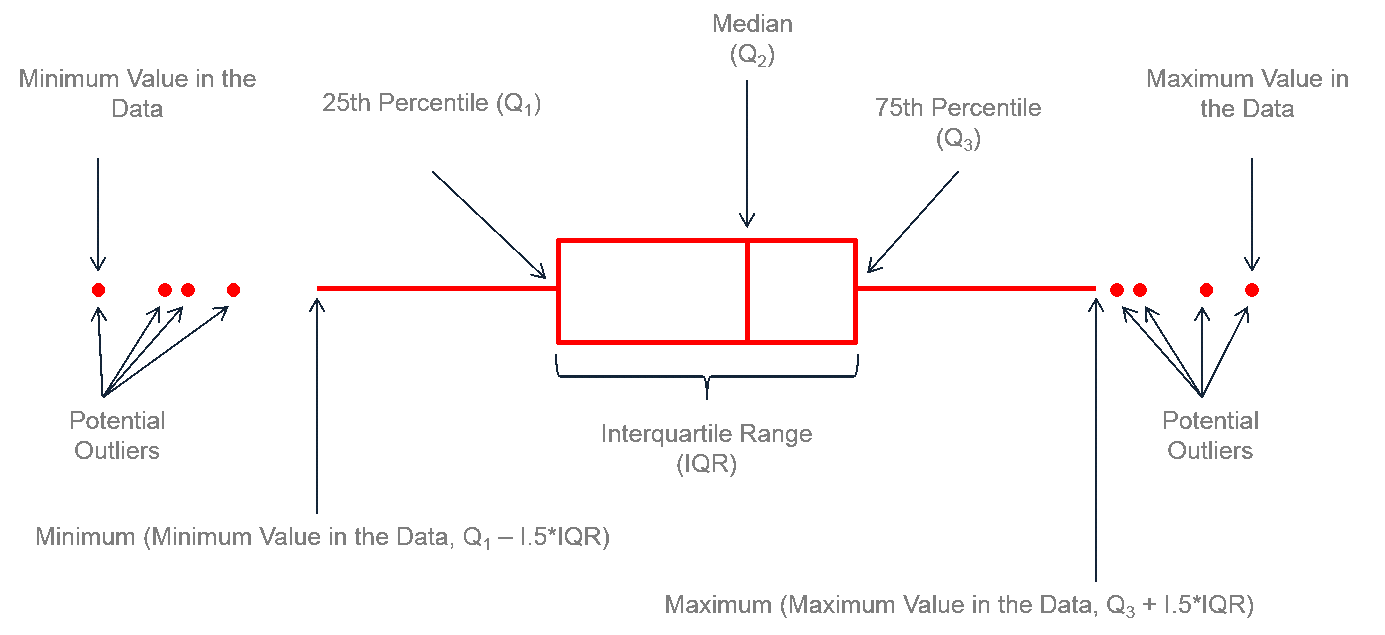Below, you can see how a dotplot representation (each dot is a cell) and the corresponding boxplot.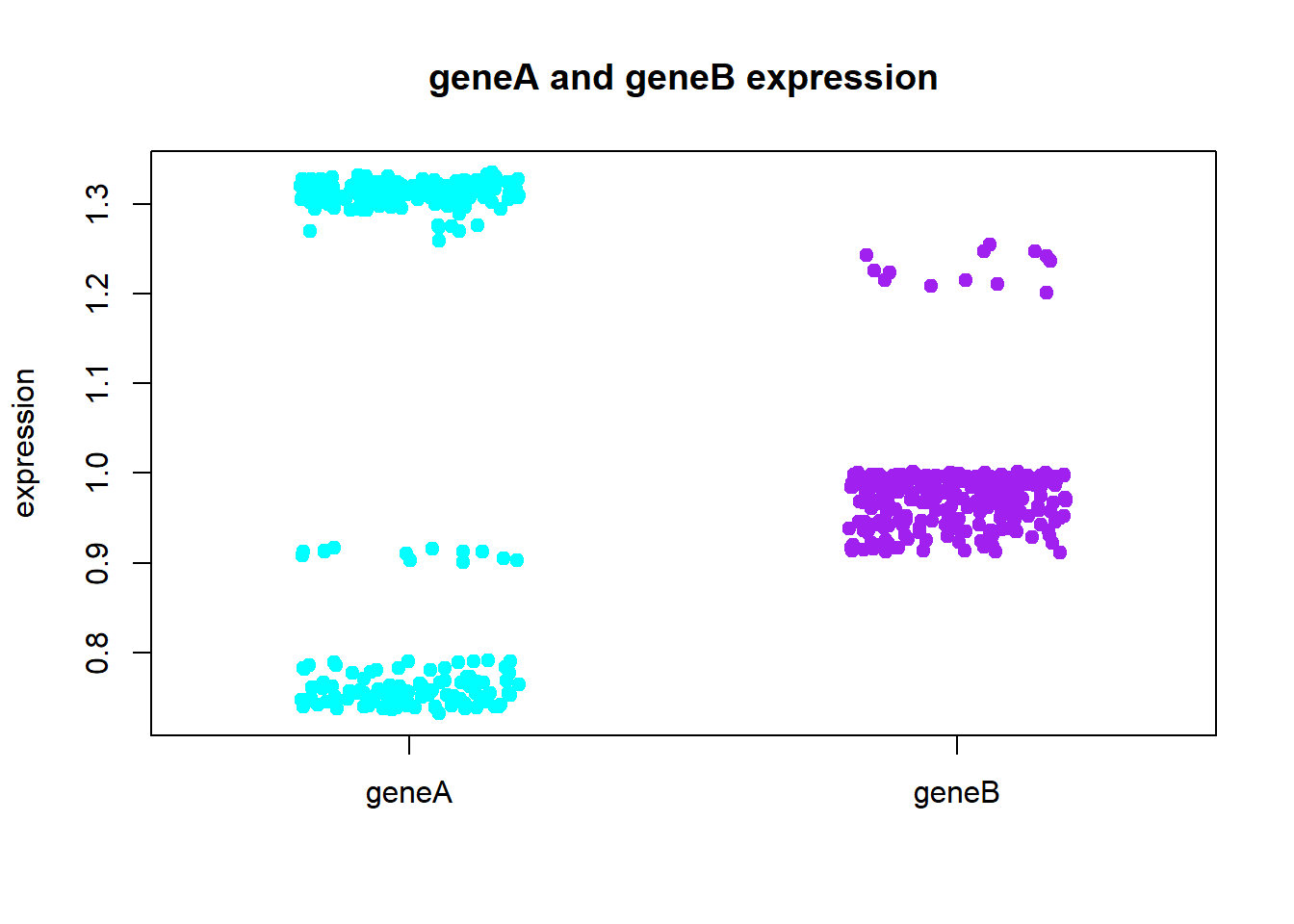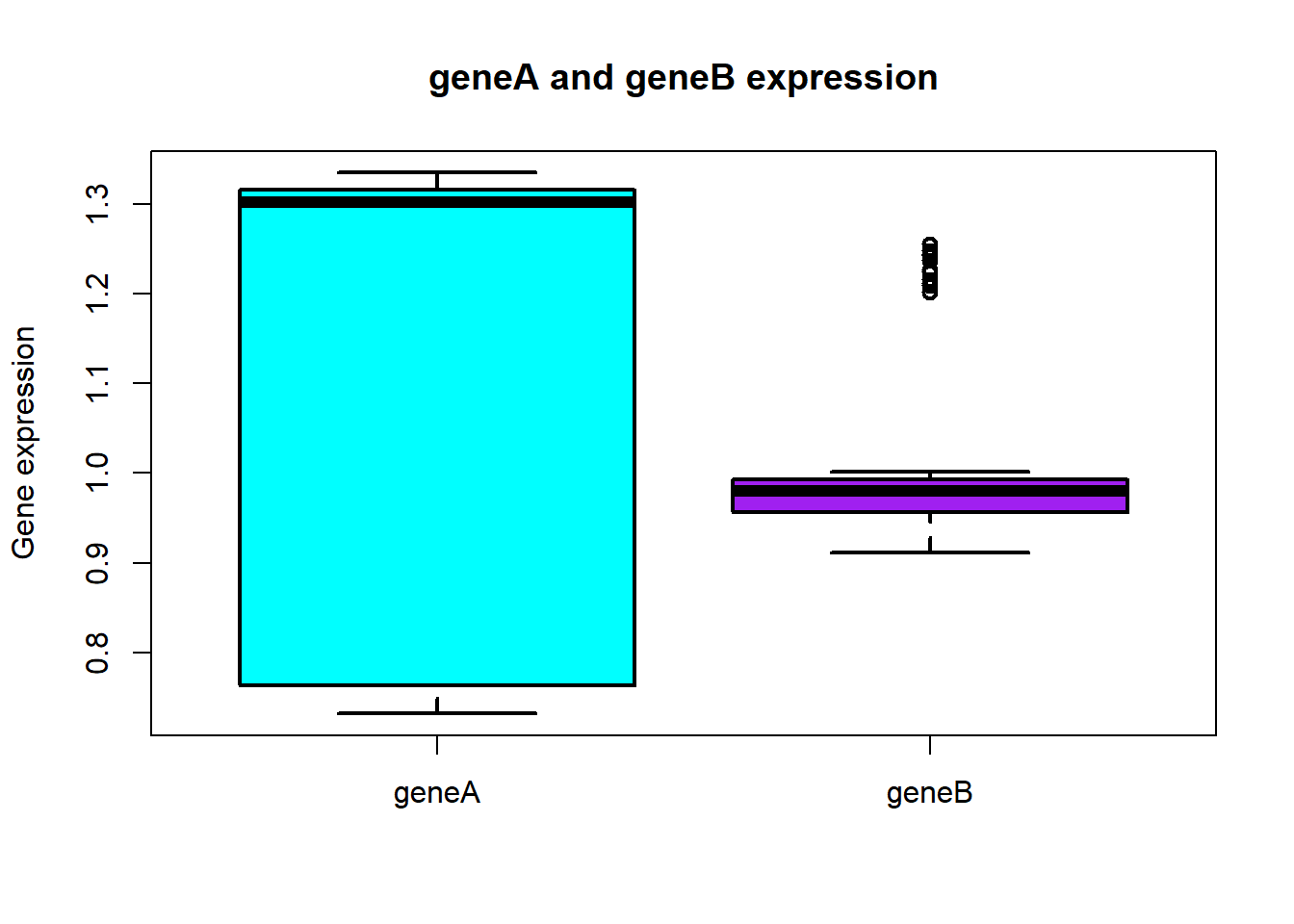The boxplot function can take as input data either a numeric vector, a list or a formula.

In R, the formula object explores a relationship between 2 or more variables. We denote this by placing a ~ (tilde) between the variables that we compare/relate. Later we will produce a boxplot geneB~cell_type which means we want to plot geneBs expression grouped by cell_type.

Below we want to do boxplots where we see each of the genes expression across all samples.

boxplot(
x=gene_data[,"geneB"], # x is a vector
col=c("purple")
)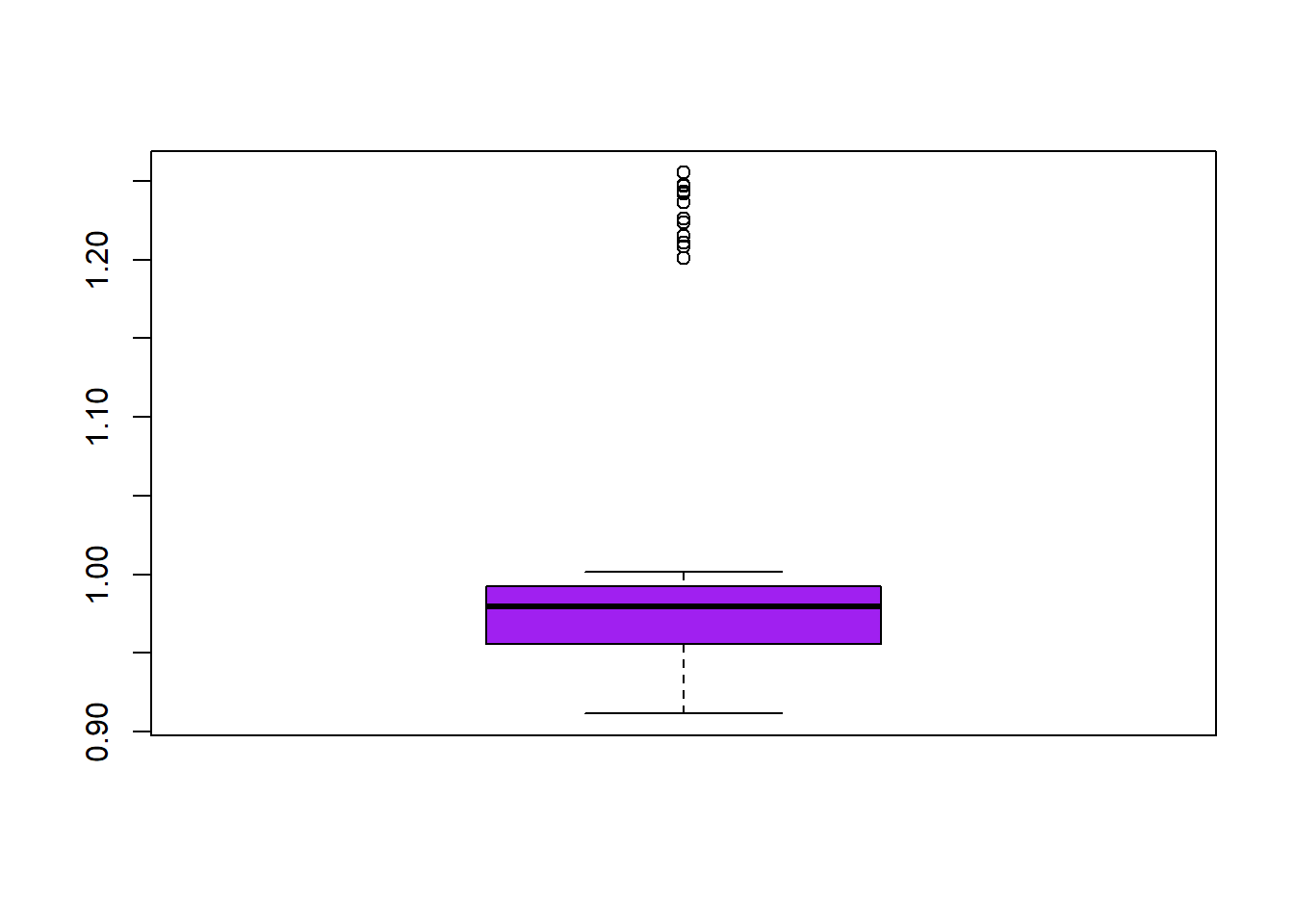boxplot(
x=list("geneA"=gene_data[,"geneA"], "geneB"=gene_data[,"geneB"]),
# x is a list with 2 elements, where each is a vector
col=c("cyan","purple")
)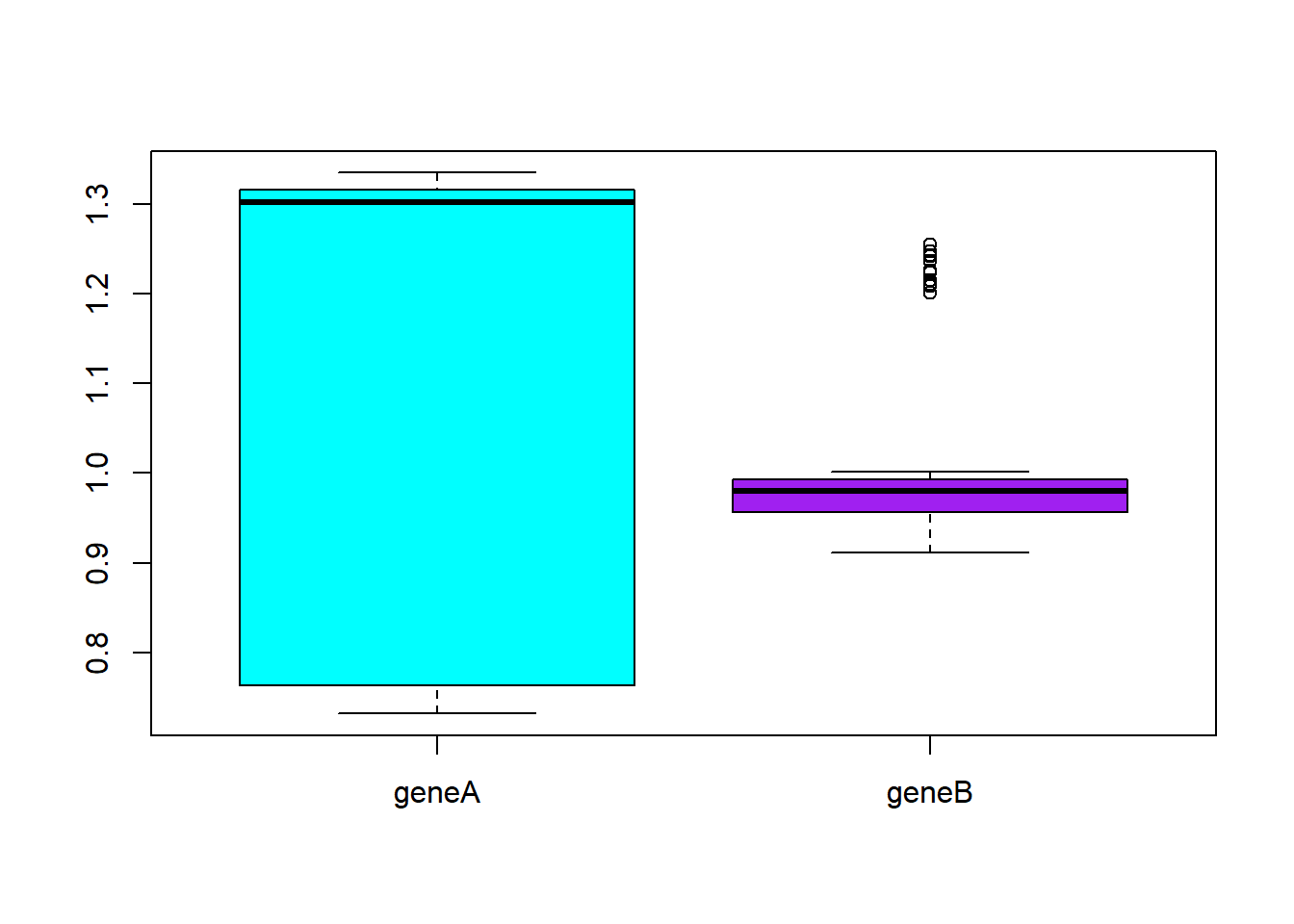# Prettifying the plot
boxplot(
x=list("geneA"=gene_data[,"geneA"], "geneB"=gene_data[,"geneB"]),
# x is a list with 2 elements
col=c("cyan","purple"),
main="geneA and geneB across all samples",
ylab="Gene expression",
xlab="Genes"
)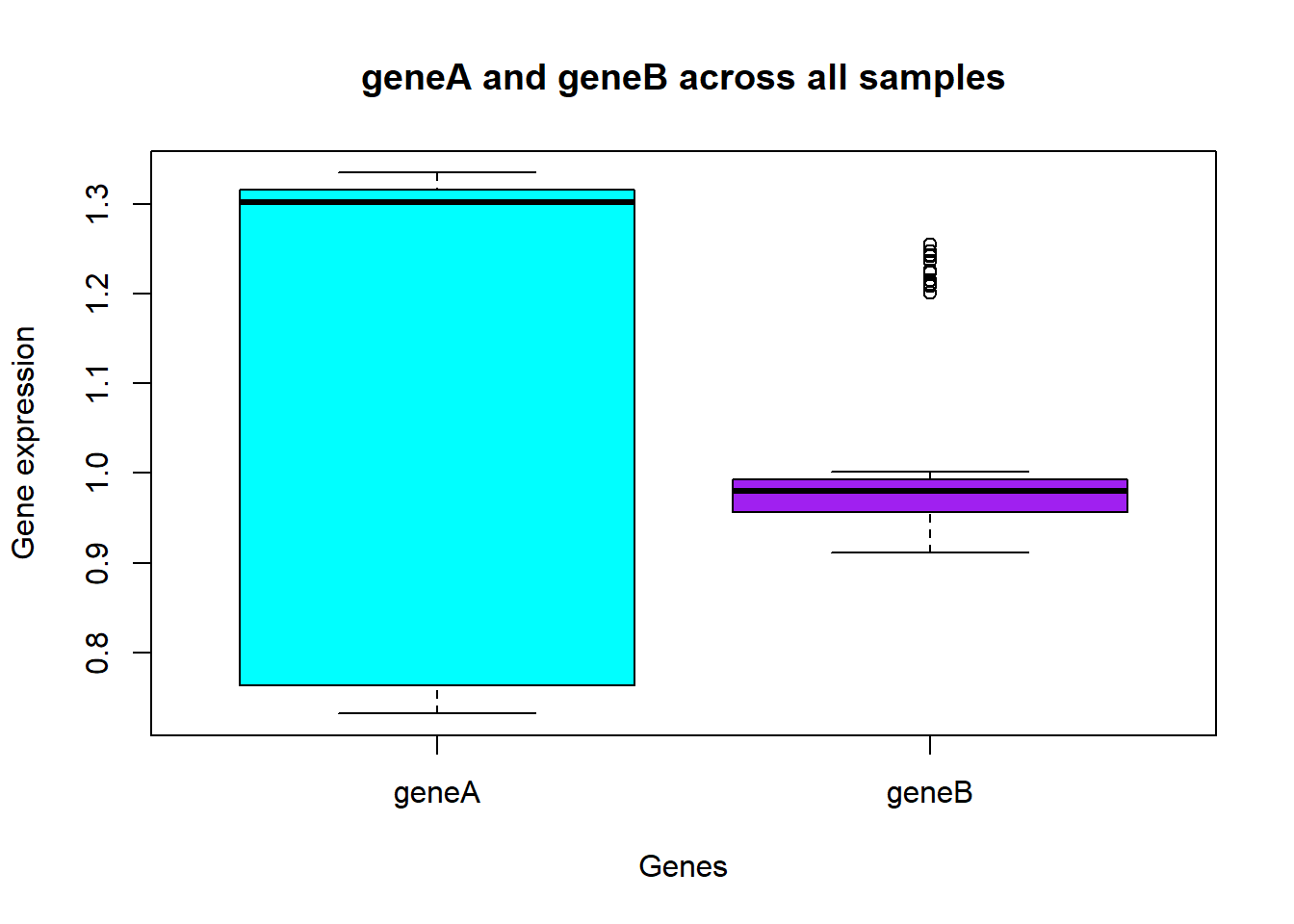Note how here we define colour differently. Do you understand why?

If you want to plot just the points without the box like in a previous example, you can use the function stripchart.

stripchart(
x=gene_data[,"geneB"],
col="purple",
vertical=TRUE,
method="jitter"
)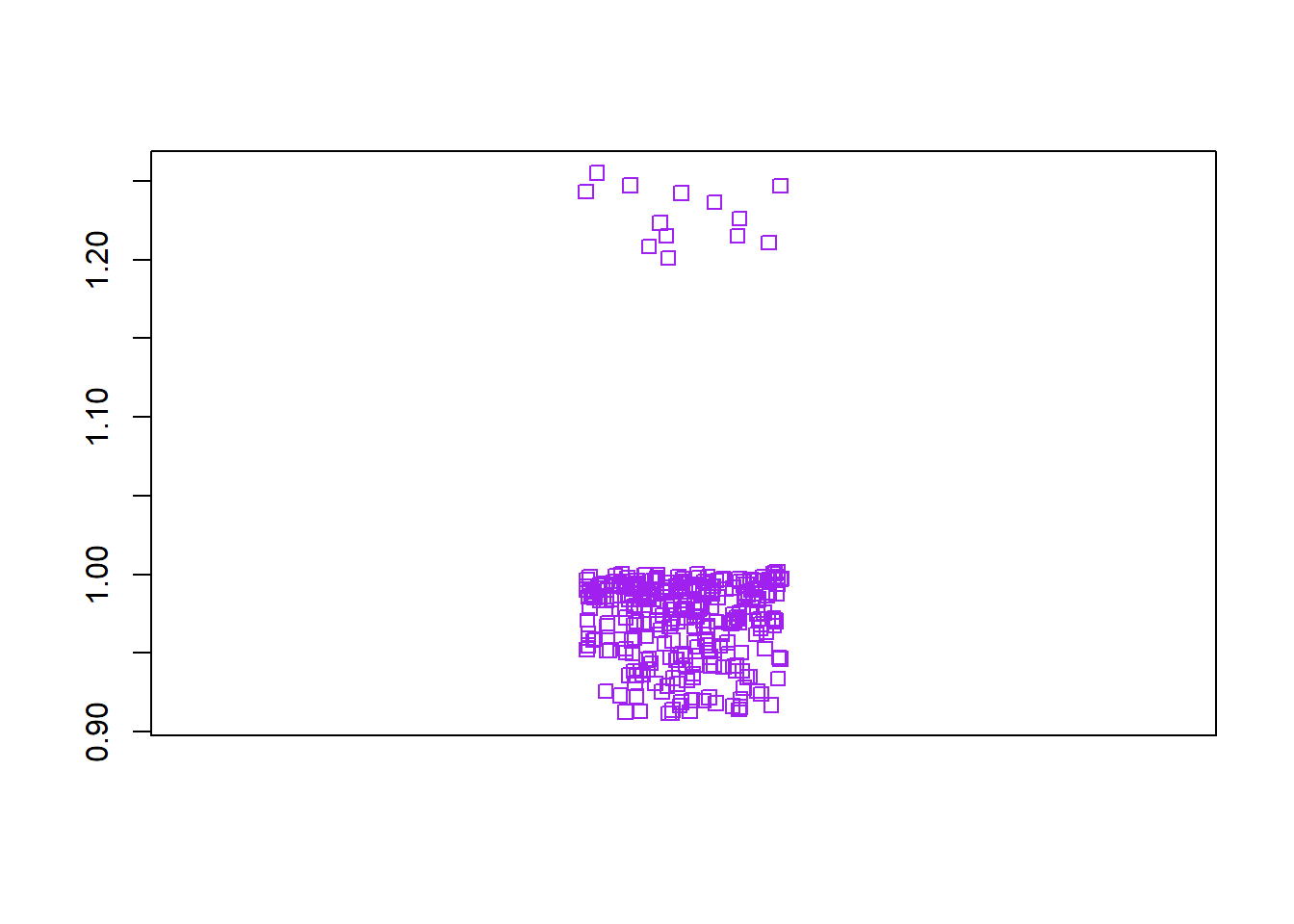Now, instead, we want to plot gene B distribution across the different cell types. Here we use formula as we are interested to contrast the expression of geneB with celltype (geneB~celltype) Since we are plotting the different cell types as before, we can use the same colour vector as before (cell_colours).

boxplot(
formula=gene_data[,"geneB"]~gene_data[,"cell_type"],
col=cell_colours
)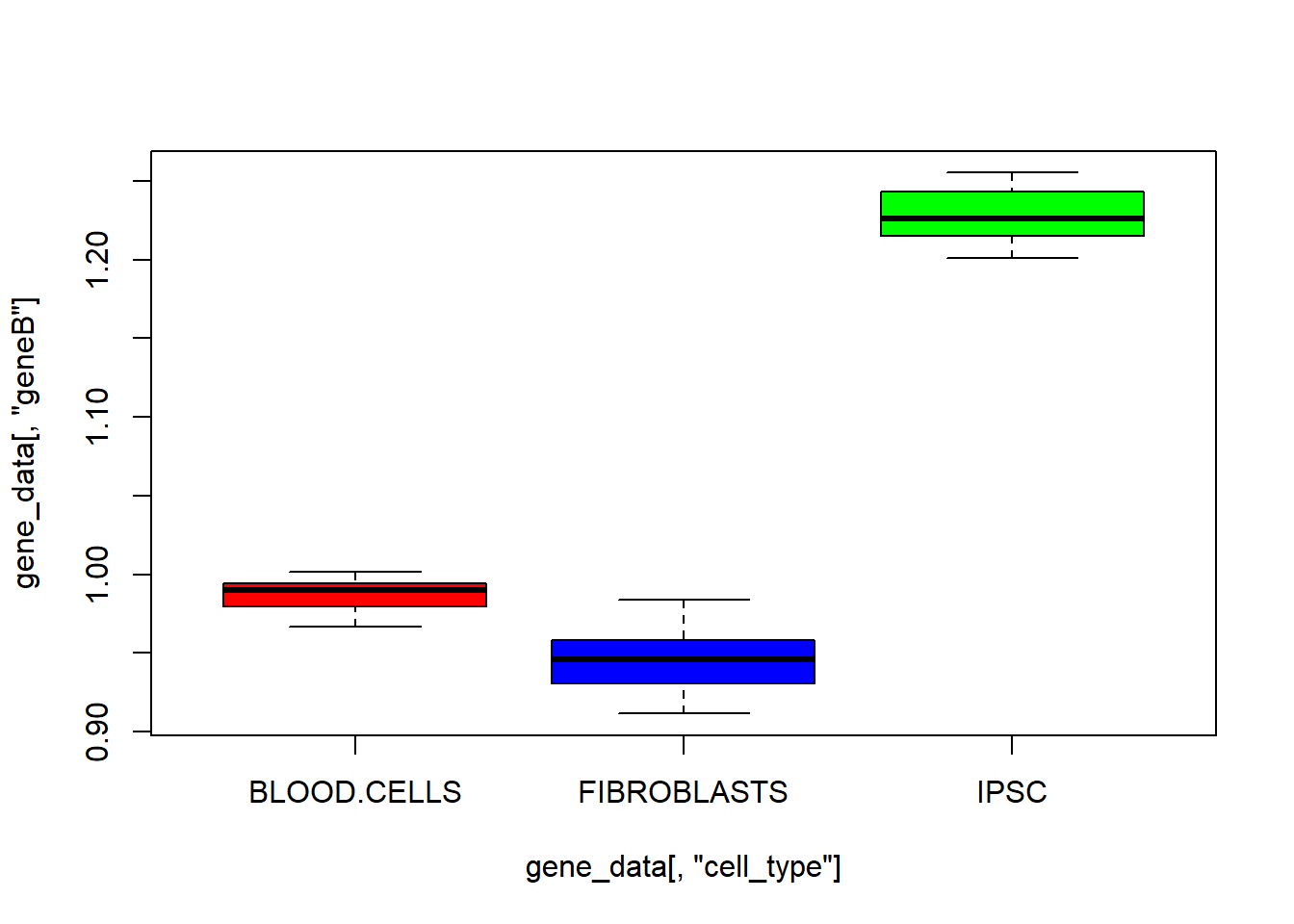# Prettifying the plot
boxplot(
formula=gene_data[,"geneB"]~gene_data[,"cell_type"],
col=cell_colours,
main="geneB expression by cell type",
ylab="Gene expression",
xlab="Cell types"
)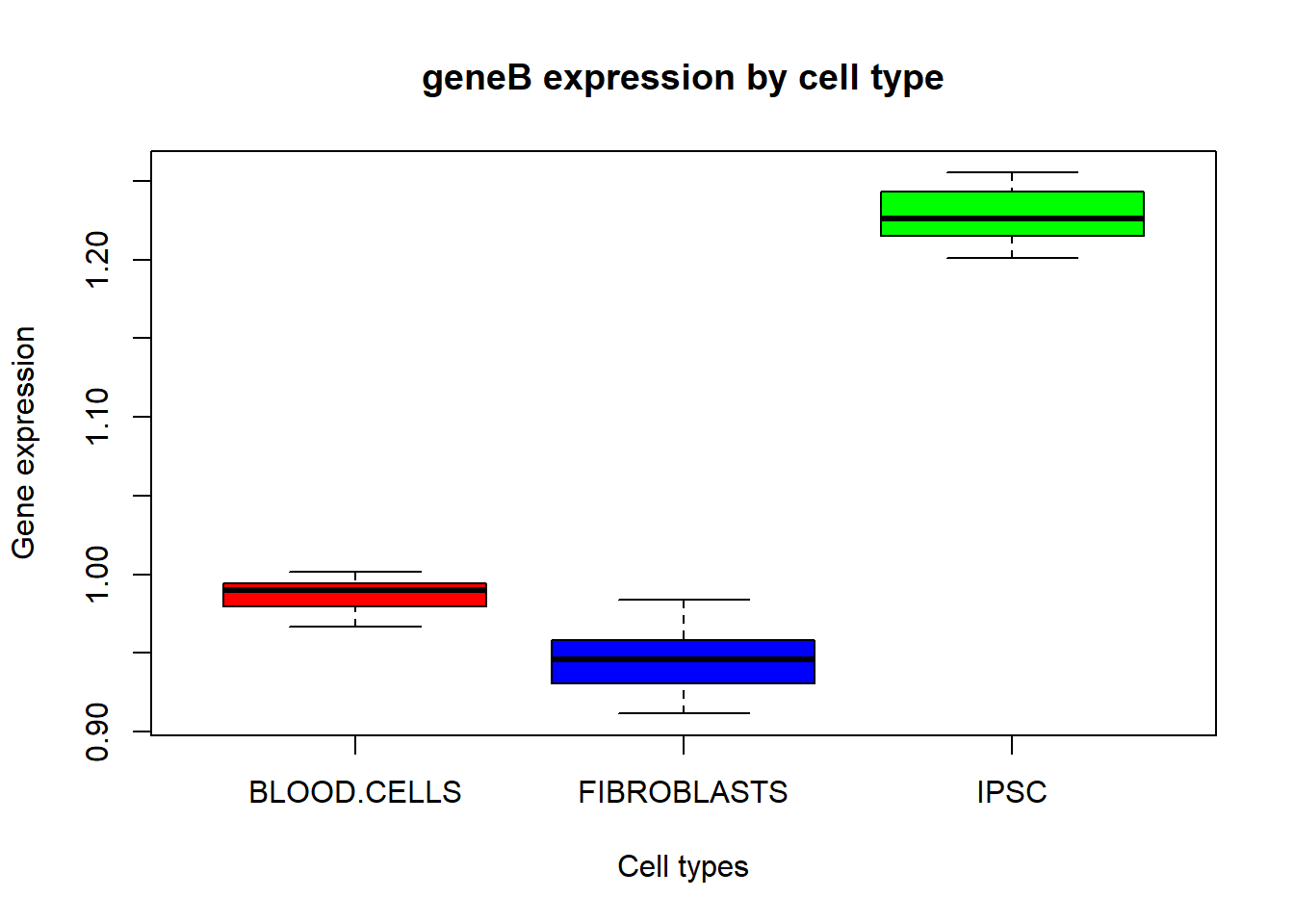# Comparing distributions

At this point you have seen boxplots with different distributions and you can, by eye, tell that they are different from each other. Running a statistical test can tell us the statistical significance of these differences.

We will show here how to use a $$t$$-test, which is only able to compare two conditions at a time. First, we need to select the column with geneB data. We will save the result to a variable.

# Substting geneB
geneB_data = gene_data[,"geneB"]

We can do the same for the cell_type column.

# Substting geneB
cell_type_data = gene_data[,"cell_type"]

Now, using logical operators we can further subset geneB data with the cell types we want to compare.

# Substting geneB with different cell types
bloodcell_geneB_data = geneB_data[cell_type_data=="BLOOD.CELLS"]
fibroblasts_geneB_data = geneB_data[cell_type_data=="FIBROBLASTS"]
ipsc_geneB_data = geneB_data[cell_type_data=="IPSC"]

With the different subsets of data ready to be compared we can run the $$t$$-test.

# t-test
res_blood_fibro = t.test(
bloodcell_geneB_data,
fibroblasts_geneB_data
)

res_ipsc_fibro = t.test(
ipsc_geneB_data,
fibroblasts_geneB_data
)

res_ipsc_blood = t.test(
ipsc_geneB_data,
bloodcell_geneB_data
)

res_blood_fibro
##
##  Welch Two Sample t-test
##
## data:  bloodcell_geneB_data and fibroblasts_geneB_data
## t = 22.91, df = 143.26, p-value < 2.2e-16
## alternative hypothesis: true difference in means is not equal to 0
## 95 percent confidence interval:
##  0.03921498 0.04662096
## sample estimates:
## mean of x mean of y
## 0.9871546 0.9442366
res_ipsc_fibro
##
##  Welch Two Sample t-test
##
## data:  ipsc_geneB_data and fibroblasts_geneB_data
## t = 54.683, df = 15.167, p-value < 2.2e-16
## alternative hypothesis: true difference in means is not equal to 0
## 95 percent confidence interval:
##  0.2732381 0.2953807
## sample estimates:
## mean of x mean of y
## 1.2285460 0.9442366
res_ipsc_blood
##
##  Welch Two Sample t-test
##
## data:  ipsc_geneB_data and bloodcell_geneB_data
## t = 48.744, df = 12.505, p-value = 1.212e-15
## alternative hypothesis: true difference in means is not equal to 0
## 95 percent confidence interval:
##  0.2306497 0.2521332
## sample estimates:
## mean of x mean of y
## 1.2285460 0.9871546

We can see from the output produced that all tests came back as significant (p-value < 0.05). Still, since the output generated is a complex object, it is interesting to explore it a bit. Using the function str we can see relevant information about the object.

str(res_ipsc_blood )
## List of 10
##  $statistic : Named num 48.7 ## ..- attr(*, "names")= chr "t" ##$ parameter  : Named num 12.5
##   ..- attr(*, "names")= chr "df"
##  $p.value : num 1.21e-15 ##$ conf.int   : num [1:2] 0.231 0.252
##   ..- attr(*, "conf.level")= num 0.95
##  $estimate : Named num [1:2] 1.229 0.987 ## ..- attr(*, "names")= chr [1:2] "mean of x" "mean of y" ##$ null.value : Named num 0
##   ..- attr(*, "names")= chr "difference in means"
##  $stderr : num 0.00495 ##$ alternative: chr "two.sided"
##  $method : chr "Welch Two Sample t-test" ##$ data.name  : chr "ipsc_geneB_data and bloodcell_geneB_data"
##  - attr(*, "class")= chr "htest"

We can see it is a list with 10 elements. The 2 most interesting elements to look at are probably statistic and p.value. Since the elements in the list have names, we can use these names to select these elements.

res_ipsc_blood["statistic"]
## $statistic ## t ## 48.7443 res_ipsc_blood["p.value"] ##$p.value
##  1.211693e-15

statistic gives us the value of the t-score, while p.value gives us the p-value for the test. Generally a large t-score indicates that the 2 groups are different while a small t-score indicates that the 2 groups are similar.

For the p-value, given an interval of confidence of 95% and an alternative hypothesis that the 2 groups have different mean distributions, a p-value < 0.05 tells us that this difference did not occur by chance and that, therefore, this difference is significant.

# Exercise

Now you are going to try and run the same analysis but using a different dataset with different cells instead.

Open the file gene_expression_data2.csv in Excel. Observe it a bit, then load the file into R and go from there.

You should create (at least):

• A pie chart with the cell type constitution.
• A scatter plot where each axis is the gene expression of the 2 genes in that dataset.
• A boxplot with both of the genes expression distribution across all samples.
• A boxplot depicting each genes expression distribution for the different cell types.
• A t-test comparing gene expression between different cell types.

# Level 6

We now take a look at Loops in R.

Loops are used to do the same task repately. Like functions, loops have a head (round brackets) and a body (curly brackets).

The for-loop is the most basic loop and looks like this:

for(i in 1:10){print(i^2)}
##  1
##  4
##  9
##  16
##  25
##  36
##  49
##  64
##  81
##  100

As an output we get the numbers from 1 to 10 squared. But what happens inside the loop? i (often used, short for index) is a variable defined in the head of the function. And as the loop progresses, i increases by 1 in every step. And in each step the body of the function is newly evaluated. Of course you can name this variable as you want. It is strongly encouraged to use speaking names in your own loops and functions, as in half a year you probably won’t remember what i,j,k where.

A for-loop can also loop through a vector

vec = c(1,3,6)
for(i in 1:length(vec)){print(vec[i]^2)}
##  1
##  9
##  36

The command length gives us the length of the vector (in this case 3). Our i is now the index of an element of the vector. e.g. vec,vec,vec. And the element on this position is then used in the body of the loop.

Another way to write this

for(i in vec){print(i^2)}
##  1
##  9
##  36

Here we take directly the elements of the vector instead of indices.

Another kind of loop is the while loop. Here we define a condition in the head which needs to be true, so that the body of the function can be executed

i = 1
while(i <= 10){
print(i^2)
i = i + 1 # Although mathematically unsolvable, in R it just overwrites the variable to the left, with the value from the right.
}
##  1
##  4
##  9
##  16
##  25
##  36
##  49
##  64
##  81
##  100

Note that we have to add +1 to i in every iteration, otherwise the statement i <= 10 would be true forever and we would have created an infinite loop.

Now we want to combine loops and functions. We want to write a function, that takes a vector of any length and computes the sum, without using the pre-defined function sum.

mySum=function(vec){ # vec is just a placeholder variable for any vector
# Create a new empty vector, to store the elements of the old vecotr
sum = 0
# 'i' iterates over the elements of the input vector
for(i in vec){
sum = sum + i
}
return(sum)
}

Or iterate over the length of the vector

mySum=function(vec){
sum = 0
# 'i' iterrates over the length of the vector
for(i in 1:length(vec)){
sum = sum + vec[i] # We now take the index of the vector
}
return(sum)
}

You can try it out by calling mySum with any vector that you can think of and check the results manually.

# Exercise

Write a function in R, using loops, that

• Produces a vector containing the median for every column of any n x m matrix

• Takes the sum of all elements of any n x m matrix WITHOUT using sum, colSums or rowSums Hints: You should use two loops. One for the rows and one for the columns. Notice that the variables in the function heads should be named differently (e.g. i and j). Check out the two functions ncol (number of columns) and nrow (number of rows)